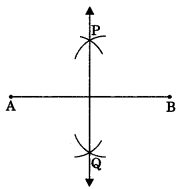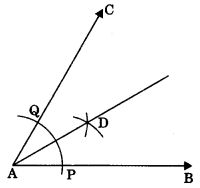On this page, you will find Constructions Class 9 Notes Maths Chapter 12 Pdf free download. CBSE NCERT Class 9 Maths Notes Chapter 12 Constructions will seemingly help them to revise the important concepts in less time.

## CBSE Class 9 Maths Chapter 12 Notes Constructions

### Constructions Class 9 Notes Understanding the Lesson

• Geometrical construction means using only a ruler and a pair of compasses as geometrical instruments.
• Protractor may be used for drawing non-standard angles.

Construction of bisector of a line segment using compass

• Draw a line segment, say AB.
• With both the end points (A and B) of line segment as centre and taking radius of more than half of the measure of line segment, draw the arcs on both sides, which cut at two points on opposite side, say P and Q.• Join these two points.
This line (PQ) is the required bisector.

Construction of the bisector of a given angle (say ∠ABC)

• With A as centre and a small radius draw an arc, cutting AB at P and AC at Q.
• With P as centre and the same radius as above, draw an arc.
• With Q as centre and with same radius, draw another arc, cutting the previous arc at D.